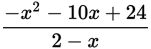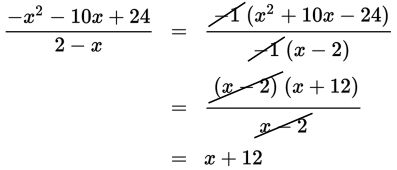# SAT Math Multiple Choice Question 533: Answer and Explanation

### Test Information

Question: 533

8.Which of the following is equivalent to the expression above, given that x ≠ 2?

• A. –x – 12
• B. x – 12
• C. 12 – x
• D. x + 12

Explanation:

D

Difficulty: Hard

Category: Passport to Advanced Math / Exponents

Strategic Advice: You could use polynomial long division to answer this question, or you could try to factor the numerator and see if any terms cancel. It is very tricky to factor a quadratic equation with a negative coefficient on x2, so start by factoring –1 out of both the numerator and the denominator.

Getting to the Answer: To factor the resulting quadratic in the numerator, you need to find two numbers whose product is –24 and whose sum is 10. The numbers are –2 and +12.